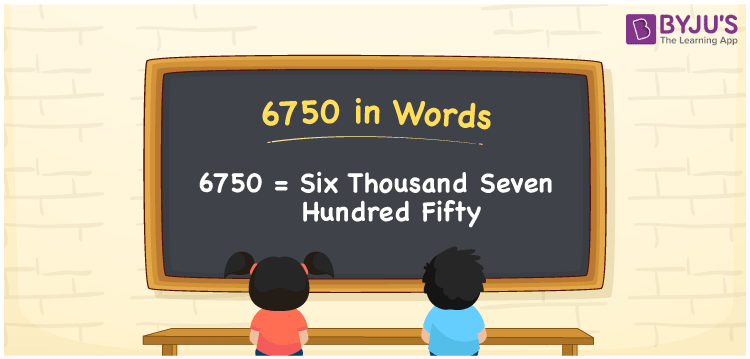# 6750 in Words

We can write 6750 in words as Six thousand seven hundred fifty. If you bought a new pair of hoodies at Rs. 6750, then you can say, “I bought a new pair of hoodies for Six thousand seven hundred fifty rupees”. That means we use the word form of cardinal numbers to express a certain amount or numerical quantity. In this article, you will learn to convert the number 6750 into words in an easily understandable method.

 6750 in words Six thousand seven hundred fifty Six thousand seven hundred fifty in Numbers 6750

## 6750 in English Words

We generally express numbers in words using the English alphabet. So, we can spell 6750 in English as “Six thousand seven hundred fifty”.## How to Write 6750 in Words?

The number 6750 contains four digits, so we need to make a place value chart with four columns to define the place values for all the digits. This can be done as given below.

 Thousands Hundreds Tens Ones 6 7 5 0

Here, ones = 0, tens = 5, hundreds = 7, and thousands = 6.

Thus, we can write the expanded form for the above digits as:

6 × Thousand + 7 × Hundred + 5 × Ten + 0 × One

= 6 × 1000 + 7 × 100 + 5 × 10 + 0 × 1

= 6000 + 700 + 50

= Six thousand + Seven hundred + Fifty

= Six thousand seven hundred fifty

Therefore, 6750 in words = Six thousand seven hundred fifty.

6750 is a natural number that precedes 6751 and succeeds 6749.

6750 in words – Six thousand seven hundred fifty

Is 6750 an odd number? – No

Is 6750 an even number? – Yes

Is 6750 a prime number? – No

Is 6750 a composite number? – Yes

Is 6750 a perfect square number? – No

Is 6750 a perfect cube number? – No

## Frequently Asked Questions on 6750 in Words

Q1

### How do you write 6750 in words?

The number 6750 can be written in words as Six thousand seven hundred fifty.
Q2

### How to write Rs. 6750 in words on a cheque?

On a cheque, we can write an amount of Rs. 6750 in words as “Six thousand seven hundred fifty rupees only”.
Q3

### How do you convert 6750 into words?

We can convert the number 6750 into words by expanding the digits. This can be done as: 6750 = 6000 + 700 + 50 = Six thousand seven hundred fifty